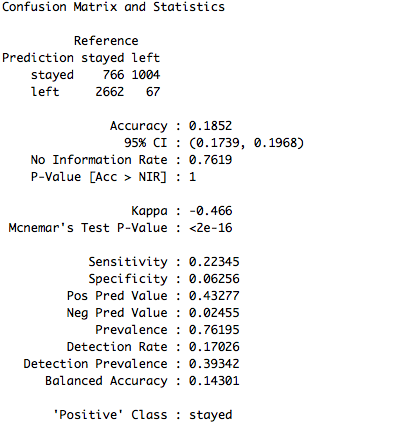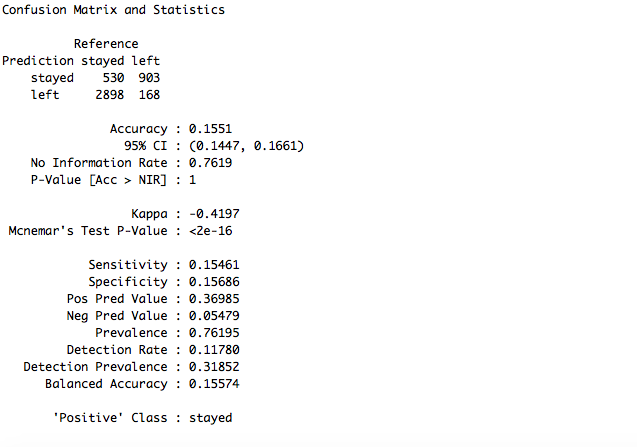{{announcement.body}}
{{announcement.title}}

# Develop Custom Ensemble Models Using Caret in R

DZone 's Guide to

# Develop Custom Ensemble Models Using Caret in R

### Here we review some different ways to create ensemble learning models and compare the accuracy of their results, seeing how each functions better as a composite.

· AI Zone ·
Free Resource

Comment (0)

Save
{{ articles.views | formatCount}} Views

Ensembling models is a robust approach to improving the accuracy of the predictive models. It is a well-thought approach very closely related to a power-packed word — TEAM !! Any work done by a team leads to significant achievements.

Likewise, in the Machine Learning world, ensemble models is a "team of models" working together to improve the result of their work. Technically, ensemble models are composed of several supervised learning models that are independently trained, and the results are combined in different ways to obtain the final prediction result. The result has better predictive performance than the results of any of its constituent learning algorithms separately. Mostly there are three types of ensemble learning methods that are generally used as described below.

## Bagging

Also known as bootstrap aggregation, it is the way to decrease the variance error of a model’s result. Sometimes the weak learning algorithms are unstable — a slightly different input leads to very different outputs. Random forest reduces this instability by running multiple instances which leads to lower variance. In this method, random samples are prepared from training data set using the bootstrapping process (random sample with replacement models). Models are built on each sample using supervised learning methods. Finally, the results are combined by averaging the results or selecting the best prediction using majority voting. Majority voting is a process in which the class with the largest number of predictions in all of the classifiers becomes the prediction of the ensemble. Similarly, there are various other approaches such as weighing and rank averaging. It's worth reading the Kaggle ensembling guide to know more about the winning strategies for developing the ensemble models.

Random forest is an example of the bagging method of ensemble models, and we will use caret in R to demonstrate it. Caret (Classification and Regression Trees) and caretEnsemble packages in R provides easy to use interface to use the seeded ensemble algorithms as well as create custom ensemble models. It is an excellent tool for R programmers, and one can use this to create almost every type of models using this package. You have methods to preprocess the data, handle class imbalance and compare results of different models with ease. You can see the caret documentation to get familiar with the caret package and acquire a convenient tool for creating a model. caretEnsemble is a package used to create ensembles of caret models.

``````# Load caret libraries
library(caret)
library(caretEnsemble)

hr_data\$left <- as.factor(hr_data\$left)
levels(hr_data\$left) <- c("stayed", "left")

# Create train and test data sets
trainIndex = createDataPartition(hr_data\$left, p=0.7, list=FALSE,times=1)

train_set = hr_data[trainIndex,]
test_set = hr_data[-trainIndex,]

seed <- 999
metric <- "Accuracy"

# Bagging Algorithm (Random Forest)
# Parameters used to control the model training process are defined in trainControl method
bagcontrol <- trainControl(sampling="rose",method="repeatedcv", number=5, repeats=3)
set.seed(seed)
#"rf" method is for training random forest  model
fit.rf <- train(left~., data=train_set, method="rf", metric=metric, trControl=bagcontrol)
# evaluate results on test set
test_set\$pred <- predict(fit.rf, newdata=test_set)
confusionMatrix(data = test_set\$pred, reference = test_set\$left)``````The accuracy obtained from the random forest model is 84.62%, which is a very decent score without any data preprocessing and feature engineering. Let's now go through another ensembling technique that is widely used to create solid models.

## Boosting

The boosting method is an iterative process in which successive models are created one after the other based on the errors of the predecessors. This helps to reduce mostly bias in the data set and somewhat leads to a reduction in variance as well. Boosting attempts to create new classifiers that are better able to predict, for which the current ensemble’s performance is weak. Unlike bagging, the resampling of the training data is dependent on the performance of the earlier classifiers. Boosting uses all data to train each classifier, but instances that were misclassified by the previous classifiers are given more weight so that subsequent classifiers improve the results.

Extreme Gradient Boosting is an example of the boosting method.

``````# Gradient Boosting
boostcontrol <- trainControl(sampling="rose",method="repeatedcv", number=5, repeats=2)
set.seed(seed)
fit.gbm <- train(left~., data=train_set, method="gbm", metric=metric, trControl=boostcontrol, verbose=FALSE)
# evaluate results on test set
test_set\$pred <- predict(fit.gbm, newdata=test_set)
confusionMatrix(data = test_set\$pred, reference = test_set\$left)``````The accuracy is 77.28% which is a little less than the accuracy obtained from random forest model. Now let's try with another ensembling approach and test its performance on the same data set.

## Stacking/Blending

In this approach, multiple layers of classifiers are stacked up one over the other. The result (i.e. probabilities) of the first layer of classifiers are used to train the second layer of classifiers and so on. The final result is obtained by using a base classifier such as logistic regression. We can use other ensemble algorithms like random forest or gbm as a final layer classifier.

In this stacked model, we will first train the model on three algorithms KNN, GLM and then rpart. The final result is obtained by using a glm model in the last layer.

``````# Stacking Algorithms
control <- trainControl(sampling="rose",method="repeatedcv", number=5, repeats=2, savePredictions=TRUE, classProbs=TRUE)
algorithmList <- c( 'knn','glm','rpart')
set.seed(seed)
stack_models <- caretList(left~., data=train_set, trControl=control, methodList=algorithmList)
stacking_results <- resamples(stack_models)
summary(stacking_results)
dotplot(stacking_results)

# Check correlation between models to ensure the results are uncorrelated and can be ensembled
modelCor(stacking_results)
splom(stacking_results)

# stack using Logistics Regression
stackControl <- trainControl(sampling="rose",method="repeatedcv", number=5, repeats=2, savePredictions=TRUE, classProbs=TRUE)
set.seed(seed)
stack.glm <- caretStack(stack_models, method="glm", metric=metric, trControl=stackControl)
print(stack.glm)
# evaluate results on test set
test_set\$pred <- predict(stack.glm, newdata=test_set)
confusionMatrix(data = test_set\$pred, reference = test_set\$left)``````The accuracy obtained is 18.52% which is way less than the other models. However, we can improve this by changing different parameters for the model and trying out different algorithms. For now, let's just switch the final layer of the stacked model to gbm and test the result.

``````# stack using gbm
set.seed(seed)
stack.gbm <- caretStack(stack_models, method="gbm", metric=metric, trControl=stackControl)
print(stack.gbm)
test_set\$pred <- predict(stack.gbm, newdata=test_set)
confusionMatrix(data = test_set\$pred, reference = test_set\$left)``````The accuracy went further down to 15.51%. Experimenting with the parameters of a model is a continuous process and one of the heavy-duty task of a Data Scientist. We will continue trying to improve the results. For now, random forest model is a clear winner.

You can find the code and dataset in my GitHub. To read more about Data Science algorithms and ensemble models, you can try out my book - Data Science Using Oracle Data Miner and Oracle R Enterprise.

Topics:
artificial intelligence ,data science ,machine learning ,r programming

Comment (0)

Save
{{ articles.views | formatCount}} Views

Opinions expressed by DZone contributors are their own.